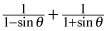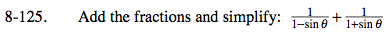### Home > PC > Chapter 8 > Lesson 8.2.4 > Problem8-125

8-125.

Add the fractions and simplify:Homework Help ✎Create a common denominator.

Combine the two fractions.

Simplify. Be sure to use the Fundamental Pythagorean Identity.

$\frac{1+\text{sin}\theta}{(1-\text{sin}\theta)(1+\text{sin}\theta)}+\frac{1-\text{sin}\theta}{(1-\text{sin}\theta)(1+\text{sin}\theta)}$

$\frac{1+\text{sin}\theta+1-\text{sin}\theta}{1-\text{sin}^2\theta}$